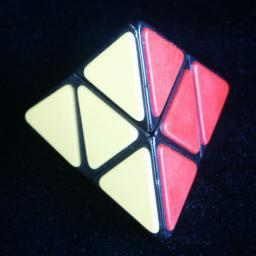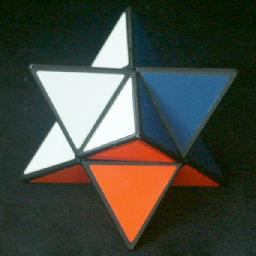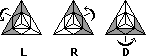# PyramorphixThe Pyramorphix puzzle is a regular tetrahedron where each face is divided into 4 smaller triangles. This puzzle is related to the 2×2×2 Rubik's Cube; it is like a cube where the pieces forming one tetrad are flat and have one colour, the other tetrad forms the vertices of the tetrahedron and have 3 colours each. This puzzle does not maintain the same shape, making it somewhat confusing to solve.

Another way to see this puzzle is as a small octahedron, on which the four faces meeting at a vertex can rotate about that vertex. Four of the faces of the octahedron have tips stuck on them, the other faces are just flat.

The Pyramorphix is currently being manufactured and sold by Uwe Meffert.

In the early eighties, this puzzle was made in East Germany under the name Figurenmatch. A patent was granted to Manfred Fritsche on 11 August 1983, DE 3,245,341. The same manufacturer also made a related puzzle called Stern, pictured above right. It is in the shape of a Stella Octangula - the pyramorphix would be obtained from this if four of its corners (the ones that only have one colour) are flattened.

If you have two spare pocket pyramorphix puzzles, you might want to take them apart and reassemble them with all the corner pieces on one puzzle and all the face pieces on the other. You now have two new puzzles, an octahedron and a Stella Octangula. It is a good idea to give them a different colour scheme.

Octahedron:
If you have four colours, two faces of each colour, then this is a really easy puzzle. There are only 8!/24/24 = 105 essentially different positions.

Stella Octangula:
This puzzle would be most interesting with 8 colours. It is then possible to end in a seemingly impossible position where one tip is twisted. In fact, it is the other three pieces of that tetrad which need to be swapped around. This puzzle has as many positions as the 2x2x2 cube, but there are four positions which can be considered solved.

## The number of positions:

There are 8 pieces, 3 orientations each, giving a maximum of 8!·38 positions. This limit is not reached because:

• The orientations of the flat pieces are not visible (34)
• the orientation of the puzzle does not matter (24)

This leaves 7!·33 = 136,080 positions.

I used a computer search to find God's Algorithm, i.e. the shortest solution for each position, and the result is shown in the table below. It shows that any position can be solved in at most 9 moves (6.6337 on average) if a half turn is considered a single move and 11 moves (8.0529 on average) if a half turn is counted as two moves.

Face turn metric Quarterturnmetric 0 1 2 3 4 5 6 7 1 6 3 24 24 96 6 144 384 72 768 1416 9 552 3692 4380 126 3456 14640 7952 5 1044 15524 29080 2932 108 4812 26396 7972 24 180 4972 5084 24 24 132 16

In Sloane's On-Line Encyclopedia of Integer Sequences this is included as sequence A079764.

Although any solution for the 2x2x2 cube will work here, I will give different solutions that uses the peculiarities of this puzzle.

## Solution 1:

I was asked by Geert Hellings to devise a simple solution to the Pyramorphix, when he arranged for the Mikrocentrum in Eindhoven to give out advertising Pyramorphixes at a conference. The solution I had at that time (solution 2 below) was much more complicated.

Phase 1: Make a pyramid shape.

1. When the puzzle is not in pyramid shape, there must be (at least) two corner pieces next to each other.
2. Bring the other two corners together as well so that you have two pairs of corners.
3. Put there two pairs opposite each other, giving the puzzle a fairly flat shape.
4. A single quarter turn will now result in the pyramid shape.Notation:
Hold the puzzle with one corner pointing towards you (the others pointing left, right and upwards), as shown in the pictures. That front corner is considered correct, and is used as a reference to place all the other pieces around it. The letters L, R, and D are used to denote moves, clockwise quarter turns of the back-left, back-right, and back-down sections of the puzzle, shown as grey in the pictures. Half turns, which don't change the pyramid shape, are denoted by L2, R2, en D2, en counter-clockwise quarter turns by L', R', en D'.

Phase 2: Put the corners in their correct positions.
One or two half turns are all that is needed to put the corners in their correct positions. The corners probably will still need to be twisted, but we will do that later.

1. Find the corner piece that belongs at the top position. To do this, simply look at the colours on the left and right sides of the reference corner. The corner you are looking for is the only other one that also has those two colours.
2. If that corner piece is not already in the top position, an L2 or an R2 turn will put it there.
3. If the corners at the left and right positions need to swapped, then do D2. When those two corners are correct, the colour on the left side of the reference corner should occur somewhere on the left corner (and similarly the right reference colour on the right corner).

Phase 3: Put the flat pieces in their correct places.

1. Identify which colour does not occur on the front reference corner. Find the flat piece of that colour.
2. That piece belongs at the rear, directly opposite the front corner. If it is not in its correct place then:
1. Hold the puzzle so that the reference corner is still in front, but that the flat piece lies in the bottom face.
2. Do the moves D2 R2 L2 R2.
3. There are now only three flat pieces left that might not yet be solved, namely those adjacent to the front corner.
If all three are incorrect, then they need to be cycled clockwise or anti-clockwise around the front corner:
anti-clockwise: L' R D2 L R'
clockwise: R L' D2 R' L ( or do the clockwise sequence twice).
4. If only two flat pieces are incorrect, then they need to be swapped.
1. Hold the puzzle so that the two pieces lie in the left and the right face (i.e. the bottom and rear face centres are correct relative to the front reference corner).
2. Do the moves R2 L D2 R L2 D'.

Phase 4: Orient the corners.

1. Hold the puzzle such that any corner you want to twist lies at the top.
2. To turn the top corner, do one of the following:
clockwise: R L' R' L R L' R' L
anti-clockwise: L' R L R' L' R L R' ( or do the clockwise sequence twice).
3. Repeat a-b for each corner that needs twisting.

Phase 5: Orient the flat pieces. (optional)
This phase is only necessary if the orientation of the flat faces is visible. This might be the case due to advertising logos, or a grain in the stickers or tiles.

1. Hold the puzzle so that any flat piece you want to turn lies in the bottom face (and the reference corner still at the front).
2. To turn the bottom flat piece, do one of the following:
clockwise: D' R2 D R' D2 R D2 R' D R2
anti-clockwise: R2 D' R D2 R' D2 R D' R2 D ( or do the clockwise sequence twice).
3. Repeat a-b for each flat piece that needs twisting. Note that the rear flat piece (opposite the reference corner) should be automatically correct once the other flat pieces are correctly oriented.

## Solution 2:

This solution has become obsolete, given the solution above, but it remains here for completeness. Some of its sequences may be useful if you wish to speedsolve.

## Notation:

In this solution, the same notation as the cube will used. The octahedral centre of the puzzle is considered fixed in space, and its vertices are denoted by the letters L, R, F, B, U and D (Left, Right Front, Back, Up and Down). Clockwise quarter turns of a face are denoted by the appropriate letter, anti-clockwise quarter turns by the letter with an apostrophe (i.e. L', R', F', B', U' or D'). Half turns are denoted by the letter followed by a 2 (i.e. L2, R2, F2, B2, U2 or D2).

Note like the 2x2x2 cube, the moves LR' perform a rotation of the whole puzzle. Similarly FB' or UD'. Therefore moving only three adjacent faces is enough to solve it. If we only use the F, R and U faces to solve it, then the DBL corner is never moved from its place, and the other pieces are simply placed so they line up with it. The solution below will mostly use this method.

Phase 1: Get it in shape.
There are only 6 possible wrong shapes that can occur, and the pyramid shape can always be reached in at most 3 moves. This is quite easy to do, so there is no need to give details.

Phase 2: Get the tips correctly positioned.

1. Hold the puzzle so that one tip is at the DBL position. This piece is from now on considered to be correctly positioned and oriented.
2. Examine the other tips to see if they are in position relative to it.
3. If only two tips need to be swapped, a single half turn move will do this.
4. If all three tips are wrong, then swap any two of them first with a half turn and then one further swap will position them all correctly.

Phase 3: Get the tips correctly oriented

1. From the previous phase the tip at DBL is correctly oriented. Examine the other three tips to see if they need a clockwise twist (+) or an anti-clockwise one (-).
2. Look the combination up in the following table and do the appropriate move sequence. Note that you will probably have to hold the puzzle in a different orientation so make sure you still have a correctly oriented piece at the DBL corner.
1. To twist DFR+ do R'UR2FU'
2. To twist DFR- do UF'R2U'R
3. To twist DFR-, UBR+ do R'F'UR'UF
4. To twist DFR-, UBR- do U2FR2F2R'
5. To twist DFR+, UBR+ do RF2R2F'U2
6. To twist DFR-, UBR-, UFL- do FUF2RF'R2
7. To twist DFR+, UBR+, UFL+ do R2FR'F2U'F'
8. To twist DFR+, UBR-, UFL- do UF'UF'UF'
9. To twist DFR-, UBR+, UFL+ do FU'FU'FU'

Phase 4: Put the other pieces in position

1. Only the face centres have to be positioned now. This can be done in one step by using one of the following sequences:
1. To swap DFL-UFR, do R2F2U2FR2U'R2F.
2. To swap DFL-UFR and UBL-DBR, do F2R2U2R2.
3. To cycle the three faces DFL->DBR->UBL->DFL, do RU'FRFR2FU2.
4. To cycle the three faces DFL->UBL->DBR->DFL, do U2F'R2F'R'F'UR'.
5. To cycle all four faces DFL->UFR->DBR->UBL->DFL, do UR2F'R2U'.

This solution takes at most 3+2+6+8=19 moves, or even 18 because of a possible cancellation between phases 1 and 2. This is quite low for such a puzzle. It could be extended to a solution for the 2x2x2 cube, by using a phase 5 which orients the 4 remaining pieces (which takes at most 8 moves).